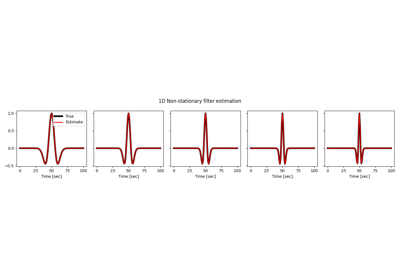# pylops.signalprocessing.NonStationaryFilters1D#

class pylops.signalprocessing.NonStationaryFilters1D(inp, hsize, ih, dtype='float64', name='C')[source]#

1D non-stationary filter estimation operator.

Estimate a non-stationary one-dimensional filter by non-stationary convolution. In forward mode, a varying compact filter on a coarser grid is on-the-fly linearly interpolated prior to being convolved with a fixed input signal. In adjoint mode, the output signal is first weighted by the fixed input signal and then spread across multiple filters (i.e., adjoint of linear interpolation).

Parameters
inpnumpy.ndarray

Fixed input signal of size $$n_x$$.

hsizeint

Size of the 1d compact filters (filters must have odd number of samples and are assumed to be centered in the middle of the filter support).

ihtuple

Indices of the locations of the filters hs in the model (and data). Note that the filters must be regularly sampled, i.e. $$dh=\text{diff}(ih)=\text{const.}$$

dtypestr, optional

Type of elements in input array.

namestr, optional

Name of operator (to be used by pylops.utils.describe.describe)

Raises
ValueError

If filters hsize is a even number

ValueError

If ih is not regularly sampled

ValueError

If ih is outside the bounds defined by dims[axis]

NonStationaryConvolve1D

1D non-stationary convolution operator.

NonStationaryFilters2D

2D non-stationary filter estimation operator.

Notes

The NonStationaryFilters1D operates in a similar fashion to the pylops.signalprocessing.NonStationaryConvolve1D operator. In practical applications, this operator shall be used when interested to estimate a 1-dimensional non-stationary filter given an input and output signal.

In forward mode, this operator uses the same implementation of the pylops.signalprocessing.NonStationaryConvolve1D, with the main difference that the role of the filters and the input signal is swapped. Nevertheless, to understand how to implement adjoint, mathematically we arrange the forward operator in a slightly different way. Assuming once again an input signal composed of $$N=5$$ samples, and filters at locations $$t_1$$ and $$t_3$$, the forward operator can be represented as follows:

$\begin{split}\mathbf{y} = \begin{bmatrix} \mathbf{X}_0 & \mathbf{X}_1 & \vdots & \mathbf{X}_4 \end{bmatrix} \mathbf{L} \begin{bmatrix} \mathbf{h}_1 \\ \mathbf{h}_3 \end{bmatrix}\end{split}$

where $$\mathbf{L}$$ is an operator that linearly interpolates the filters from the available locations to the entire input grid – i.e., $$[\hat{\mathbf{h}}_0 \quad \mathbf{h}_1 \quad \hat{\mathbf{h}}_2 \quad \mathbf{h}_3 \quad \hat{\mathbf{h}}_4]^T = \mathbf{L} [ \mathbf{h}_1 \quad \mathbf{h}_3]$$. Finally, $$\mathbf{X}_i$$ is a diagonal matrix containing the value $$x_i$$ along the main diagonal. Note that in practice the filter may be shorter than the input and output signals and the $$x_i$$ values are placed only at the effective positions of the filter along the diagonal matrices $$\mathbf{X}_i$$.

In adjoint mode, the output signal is first weighted by the fixed input signal and then spread across multiple filters (i.e., adjoint of linear interpolation) as follows

$\begin{split}\mathbf{h} = \mathbf{L}^H \begin{bmatrix} \mathbf{X}_0 \\ \mathbf{X}_1 \\ \vdots \\ \mathbf{X}_4 \end{bmatrix} \mathbf{y}\end{split}$
Attributes
shapetuple

Operator shape

explicitbool

Operator contains a matrix that can be solved explicitly (True) or not (False)

Methods

 __init__(inp, hsize, ih[, dtype, name]) adjoint() apply_columns(cols) Apply subset of columns of operator cond([uselobpcg]) Condition number of linear operator. conj() Complex conjugate operator div(y[, niter, densesolver]) Solve the linear problem $$\mathbf{y}=\mathbf{A}\mathbf{x}$$. dot(x) Matrix-matrix or matrix-vector multiplication. eigs([neigs, symmetric, niter, uselobpcg]) Most significant eigenvalues of linear operator. matmat(X) Matrix-matrix multiplication. matvec(x) Matrix-vector multiplication. reset_count() Reset counters rmatmat(X) Matrix-matrix multiplication. rmatvec(x) Adjoint matrix-vector multiplication. todense([backend]) Return dense matrix. toimag([forw, adj]) Imag operator toreal([forw, adj]) Real operator tosparse() Return sparse matrix. trace([neval, method, backend]) Trace of linear operator. transpose()

## Examples using pylops.signalprocessing.NonStationaryFilters1D#Non-stationary Filter Estimation

Non-stationary Filter Estimation